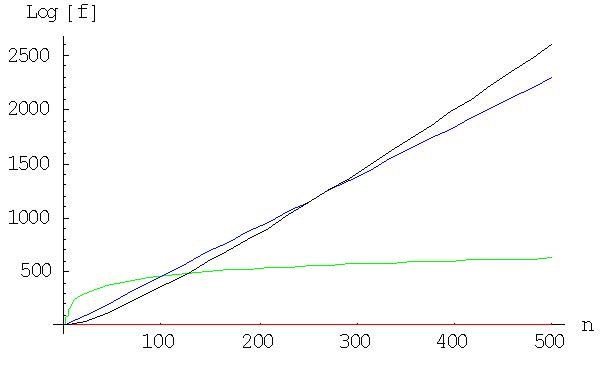#### You may also like### Climbing Powers

$2\wedge 3\wedge 4$ could be $(2^3)^4$ or $2^{(3^4)}$. Does it make any difference? For both definitions, which is bigger: $r\wedge r\wedge r\wedge r\dots$ where the powers of $r$ go on for ever, or $(r^r)^r$, where $r$ is $\sqrt{2}$?### Little and Large

A point moves around inside a rectangle. What are the least and the greatest values of the sum of the squares of the distances from the vertices?### Approximating Pi

By inscribing a circle in a square and then a square in a circle find an approximation to pi. By using a hexagon, can you improve on the approximation?

# How Does Your Function Grow?

##### Age 16 to 18Challenge Level

Here are two solutions, one from Simon from Elizabeth College, Guernsey and one from Andrei from Tudor Vianu National College, Bucharest, Romania. First Simon's solution.

The functions are: \eqalign { A(n) &= \log(100n) \cr B(n) &= n^{100} \cr C(n) &= 100^n \cr D(n) &= n! .} Firstly I looked at Archimedes' choice, the logarithmic function. $$A(n) = \log(100n) = \log 100 + \log n.$$ I knew that when $n$ gets large $\log 100$ would become irrelevant. This meant that only $\log n$ would be important, and $\log n < n$ when $n > 1$. So $A(n)$ will be less than $n$ for large $n$. We find $n = \log(100)+ \log n$ for $n = 6.472775$ approximately and so $A(n) < n$ for all values of $n> 6.5$.\par The next task was establish whether $B(n)$ or $C(n)$ was greater for large numbers. I was certain of one thing: $B(n) = C(n) = 100^{100}$ for $n=100$.

Firstly I knew that $100^n$ would have $2n+1$ digits. I then established, where $x$ is the number of digits in $n$, that $n^{100}$ would have between $100(x-1)+1$ and $100x$ digits. So $C(n) = 100^n$ is the larger function for $n> 100$.

Therefore, so far, $C(n)$ is the largest function for large values of $n$.

The next task was to compare it to $D(n)$ or factorial $n$.

Using Stirling's formula: $$D(n) = n! = \sqrt{2\pi n} ({n\over e})^n.$$ As $n$ is a large positive number, $\sqrt {2\pi n}$ will be greater than 1. Therefore when ${n\over e}$ is greater than 100, $D(n) > C(n)$. The 'change over point' occurs for $n$ just less than $n = 100e$, that is $n \approx 270$.

Andrei considered the natural logarithms of the functions and plotted their graphs. For the factorial n function Andrei used Stirling's Approximation which is valid for large n.

I approximated the function for factorial n further by ignoring the term $\sqrt{2\pi n}$. \eqalign{ A(x)&= \log (n) + \log(100) \cr \log(B(n)) &= 100 \log(n)\cr \log C(n) &= n \log(100) \cr \log D(n)&\approx n\log(n) - n }. I know that $log(n)< n$ for the whole domain of definition of the logarithmic function, and I see that $A$ is the smallest. I have to compare the other functions. As $\log(100)\approx 4.60$, so: \eqalign { \log(B) &= 100 \log(n) \cr \log(C)&\approx 4.6n \cr \log(D) &\approx n (\log(n) -1).} In the limit for large $n$, $$\lim_{n\to \infty}{\log n\over n}\to 0$$ and so $B< C$.

For large $n$ we can neglect 1 in respect to $\log(n)$ in the approximate formula for $\log(D)$ and, as $4.6 < \log (n)$ we have $D> C> B> A$.

Here in the diagram, $A(x)$ is represented in red, $B(x)$ in green, $C(x)$ in blue and $D(x)$ in black. It can be observed that for large $n$ (here $n> 300$) the order of the magnitude of the functions is $D> C> B> A$.